Dynamics of Charged Particles in Static and Uniform Electromagnetic Fields

# Dynamics of Charged Particles in Static and Uniform Electromagnetic Fields - Notes | Study Electricity & Magnetism - Physics

 Table of contentsThe Lorentz Force Law:1 Crore+ students have signed up on EduRev. Have you?

The Lorentz Force Law:

The magnetic force on a charge Q, moving with velocity v in a magnetic field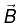is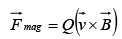This is known as Lorentz force law.
In the presence of both electric and magnetic fields, the net force on Q would be: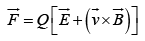Charged Particle in Static Electric Field

Charged Particle enters in the direction of field (Linear motion)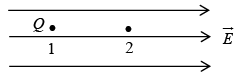The force on the charge Q in electric field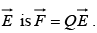Acceleration of the charge particle in the direction of the electric field is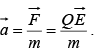If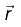is the position vector at any time t then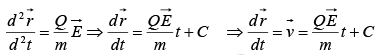where C is a constant.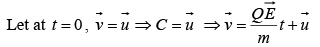Since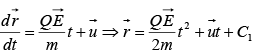where C1 is a constant

Let at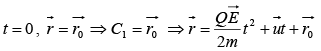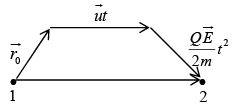If initially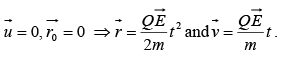The energy acquired by the charged particle in moving from point 1 to 2 is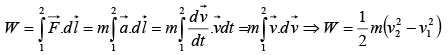If the potential difference between points 1 to 2 is V then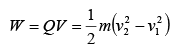If the particle starts from rest i.e (v1 = 0) and final velocity is v then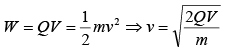Kinetic energy of the particle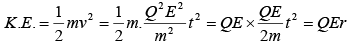Charged Particle enters in the direction perpendicular to field (Parabolic motion)
Let us consider a charge particle enters in an electric field region with velocity vx at t = 0 . The electric field is in the y-direction and the field region has length l. After traversing a distance l it strikes a point P on a screen which is placed at a distance L from the field region.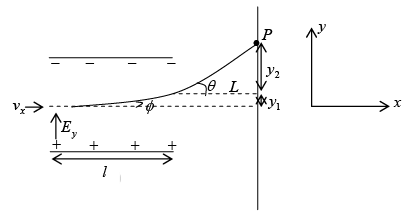Since electric field is in the y-direction, charge particle will experience force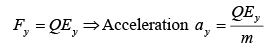In time t, charge particle will traverse a distance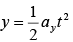in y-direction and a distance x = vxt in x-direction.

Thus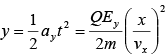and which represents parabolic path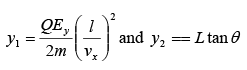Thus distance of point P from the center of the screen is,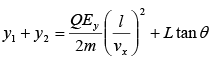Angle of deviation in the field region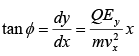Angle of deviation in the field free region,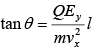Charged Particle in Static Magnetic Field

he magnetic force on a charge Q, moving with velocity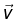in a magnetic field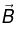is,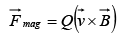This is known as Lorentz force law.

Charged Particle enters in the direction perpendicular to field (Circular motion)

If a charge particle enters in a magnetic field at angle of 90o, then motion will be circular with the magnetic force providing the centripetal acceleration. As shown in figure, a uniform magnetic field points into the page; if the charge Q moves counter clockwise, with speed v, around a circle of radius R, the magnetic force points inward, and has a fixed magnitude QvB, just right to sustain uniform circular motion: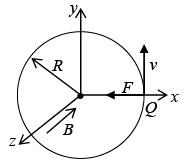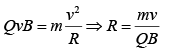where R is the radius of the circle and m is the mass of the charge particle.
Momentum of the charged particle p = QBR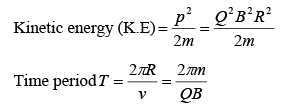Charged Particle enters in the direction making an angle with the field

(Helical motion)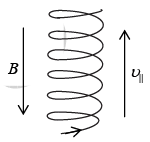If the charge particle enters in a magnetic field making an angle θ, then motion will be helical.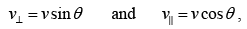and the radius of helix is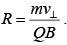Charged Particle in Uniform Electric and Magnetic Field (Cycloid motion)

If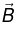points in x-direction and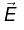points in z-direction, and a particle at rest is released from origin, then particle will follow cycloid motion.  Initially, the particle is at rest, so the magnetic force is zero, and the electric field accelerates the charge in z-direction. As it speeds up, a magnetic force develops which pulls the charge to the right. The faster it goes stronger the magnetic force becomes and it curves the particle back around towards the y-axis. At this point the charge is moving against the electric force, so it begins to slow down-the magnetic force then decreases, and the electrical force takes over, bringing the charge to rest at point a and then process  repeats.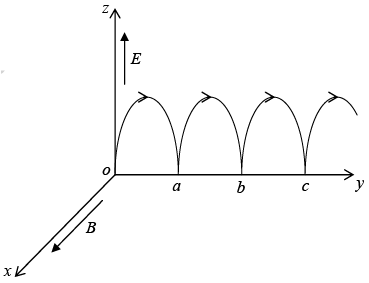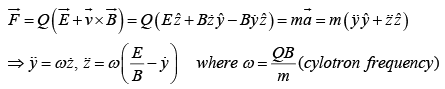Let us solve the above differential equations,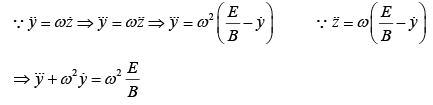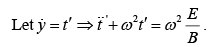For C.F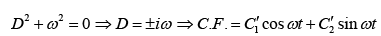For P.I.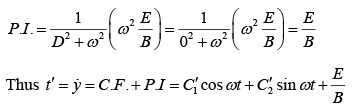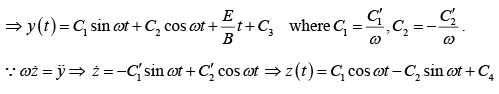Initial Condition: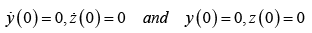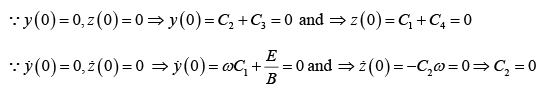Thus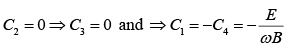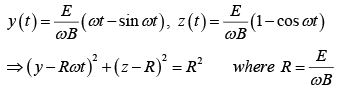This is the formula for a circle, of radius R, whose center is  ( 0, Rω t ,R ) travels in the y-direction at constant speed,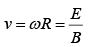The curve generated in this way is called a cycloid.
Magnetic forces do not work because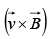is perpendicular to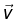so,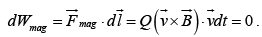Magnetic forces may alter the direction in which a particle moves, but they can not speed up or slow down it.

Example 1: A neutron, a proton, an electron and an α – particle enter a region of constant magnetic field with equal velocities. The magnetic field is along the inward normal to the plane of paper. Label the tracks of the particles.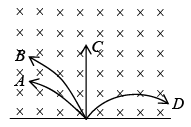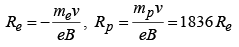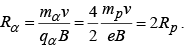A → Proton, B → α−particle, C → neutron (undeflected), D → electron.

Example 2: A uniform magnetic field with a slit system, as shown in figure, is to be used as a momentum filter for high energy charged particles. With a field B tesla, it is found that the filter transmits α–particles each of energy 5.3 MeV.
The magnetic field is increased to 2.3 B tesla and deuterons are  passed into the filter. Find the energy of each deuteron  transmitted by the filter.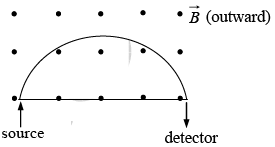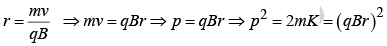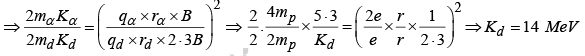Example 3: A beam of protons with velocity 4 ×105 m / sec enters a uniform magnetic field of 0.3 Tesla at an angle of 600 to the magnetic field. Find the radius of the helical path taken by the proton beam. Also find the pitch of the helix.

v = 4×105m / sec , v =v sin 60  and v|| =v cos 60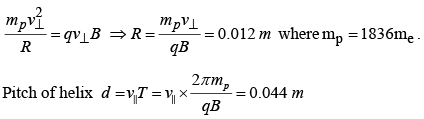The document Dynamics of Charged Particles in Static and Uniform Electromagnetic Fields - Notes | Study Electricity & Magnetism - Physics is a part of the Physics Course Electricity & Magnetism.
All you need of Physics at this link: Physics

## Electricity & Magnetism

86 videos|29 docs|22 tests

## Electricity & Magnetism

86 videos|29 docs|22 tests

Track your progress, build streaks, highlight & save important lessons and more!

,

,

,

,

,

,

,

,

,

,

,

,

,

,

,

,

,

,

,

,

,

;